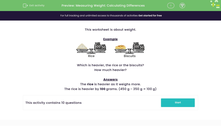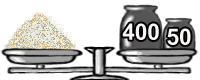# Calculate Differences in Mass

In this worksheet, students will study the masses of different foods and calculate the difference.Key stage:  KS 2

Curriculum topic:   Measurement

Curriculum subtopic:   Measure, Compare, Add/Subtract Lengths, Mass, and Volume/Capacity

Difficulty level:#### Worksheet Overview

In this activity, we will be learning to compare masses in grams.

Measuring the mass of something is a way of working out how light or heavy it is.

We often use weighing scales for this, similar to those in the pictures below.We will be comparing masses in grams and we may need to do some adding up or subtracting before we can answer the question.

You could use mental methods, or written methods to do this and it might help to jot down your calculations on paper while you are working.

Let's go ahead and try an example together, as an introduction to this activity.

Example

Which is heavier: the rice or the biscuits?

How much heavier?Rice Biscuits

First, let's find out which has the greatest mass by doing some mental calculations.

The rice has a mass of 450 grams

400 + 50 = 450 g

The biscuits have a mass of 350 grams.

300 + 50 = 350 g

So, the rice is heavier as it weighs more.

Now, we can use subtraction to work out how much heavier the rice is, compared to the biscuits.

Find the difference between the two masses: 450 g - 350 g = 100 g

The rice is heavier by 100 grams.

Does that make sense?### What is EdPlace?

We're your National Curriculum aligned online education content provider helping each child succeed in English, maths and science from year 1 to GCSE. With an EdPlace account you’ll be able to track and measure progress, helping each child achieve their best. We build confidence and attainment by personalising each child’s learning at a level that suits them.

Get started